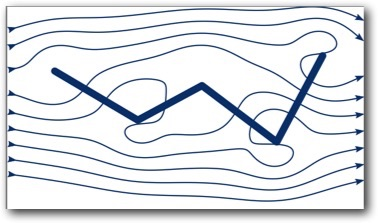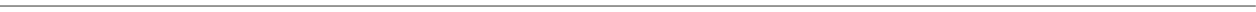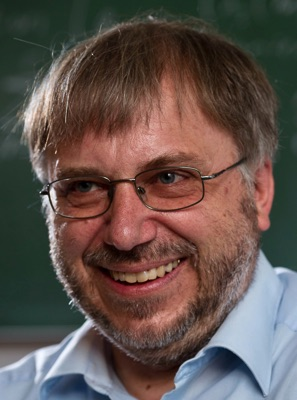Christian Klingenberg

research interests:

•  mathematical fluid mechanics

•   compressible gas dynamics, magnetohydrodynamics

•   kinetic modeling, kinetic modeling of gas mixtures and of plasma, numerics for this

•   theory of hyperbolic conservation laws: well-posedness in one and multiple space dimensions, relaxation limits, discontinuous flux conservation laws

•   modeling macroscopic fluid flow equations via microscopic interacting particle systems

•   numerics for hyperbolic conservation laws: finite volume schemes in one and multiple space dimensions, approximate Riemann solvers, discontinuous Galerkin methods, moving mesh methods

•  low Mach limits for the Euler equations and equations of ideal magnetohydrodynamics, also with gravity, both theoretical and numerical

•  well-balanced schemes applied to the Euler equations and equations of ideal magnetohydrodynamics, both with gravity

•  numerical methods that preserve stationary solutions for for kinetic and fluid equations

•  uncertainty quantification, theoretical coercivity estimates, numerical simulations for kinetic and fluid equations

•  astrophysical applications: accretion discs, protostellar jets, turbulence modeling, large eddy simulations, star formation, stellar evolution, galaxy evolutionOur logo, showing the interplay between astrophysics (Cassiopeia) and fluid mechanics.

designed together with Sebastian Ohlmann

former workshops, conferences etc.:

-  Oberwolfach workshop "Structure preserving numerical methods for hyperbolic equations", April 11 - 15, 2022 see here

-  Oberwolfach workshop "Modelling phenomena from nature by hyperbolci conservation laws", April 11, 17, 2021 see here

-      Fall school Oct. 1 - 5, 2018 in Würzburg: Hyperbolic conservation laws and mathematics of fluid mechanics, with lectures by   Constantine Dafermos, Eduard Feireisl, Sara Daneri and Simon Markfelder

-      16th International Conference on Hyperbolic Problems, organized by M. Westdickenberg and myself Aug. 1 - 5, 2016.

-  Workshop in Würzburg, June 30 - July 3, 2015,Evolutionary Equations, theory & numerics”, see hereDept. of Mathematics

Würzburg University

Emil Fischer Str. 40

97074 Würzburg

Germany

I am in room 03.011 (top floor)

phone: (0049) (0)931 318 5045

email:    klingen "at" mathematik.uni-wuerzburg.de

upcoming visitors:

- tba

ongoing Seminar Series on

Hyperbolic equations: Structure preserving numerical methods and other topics, see here